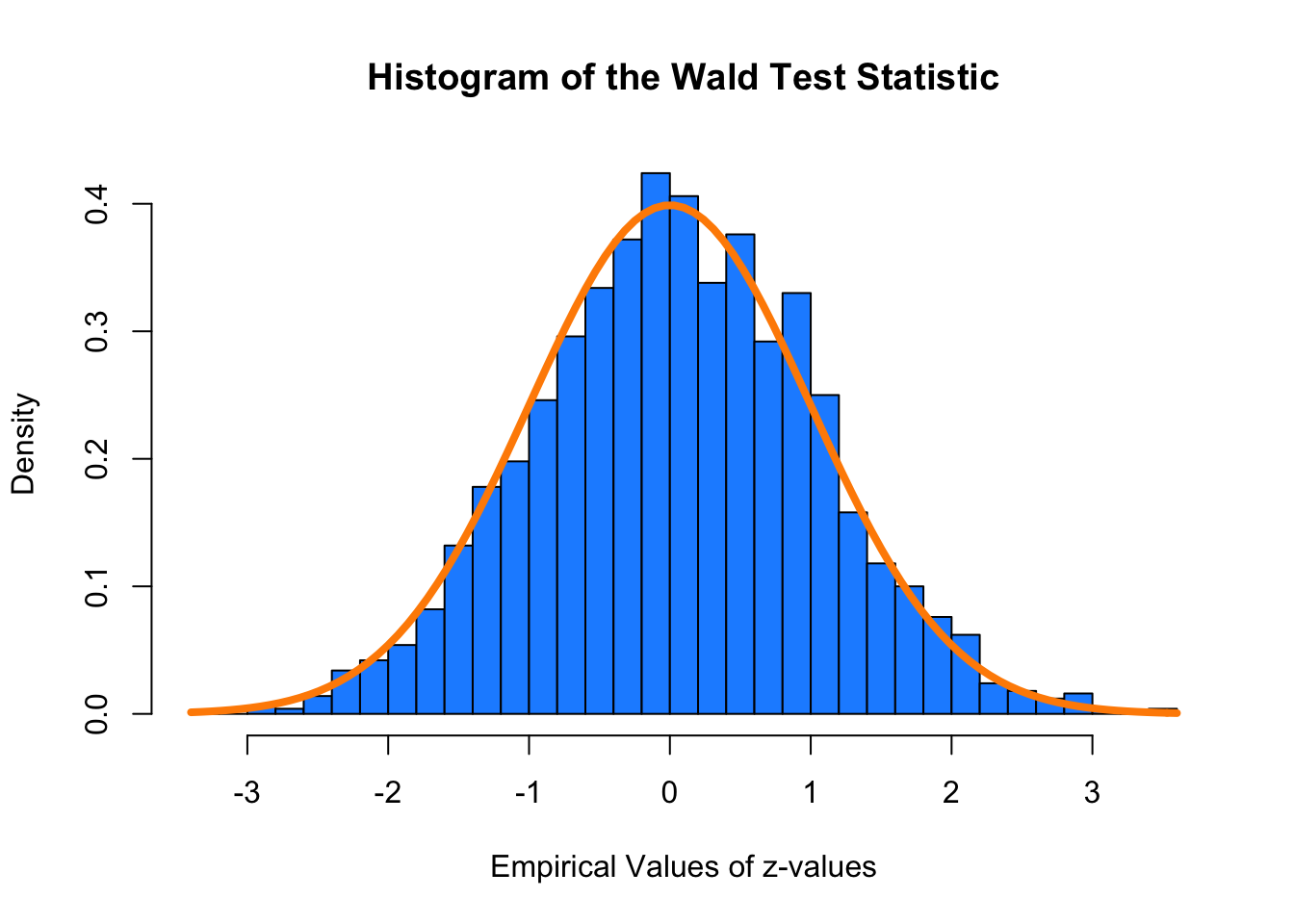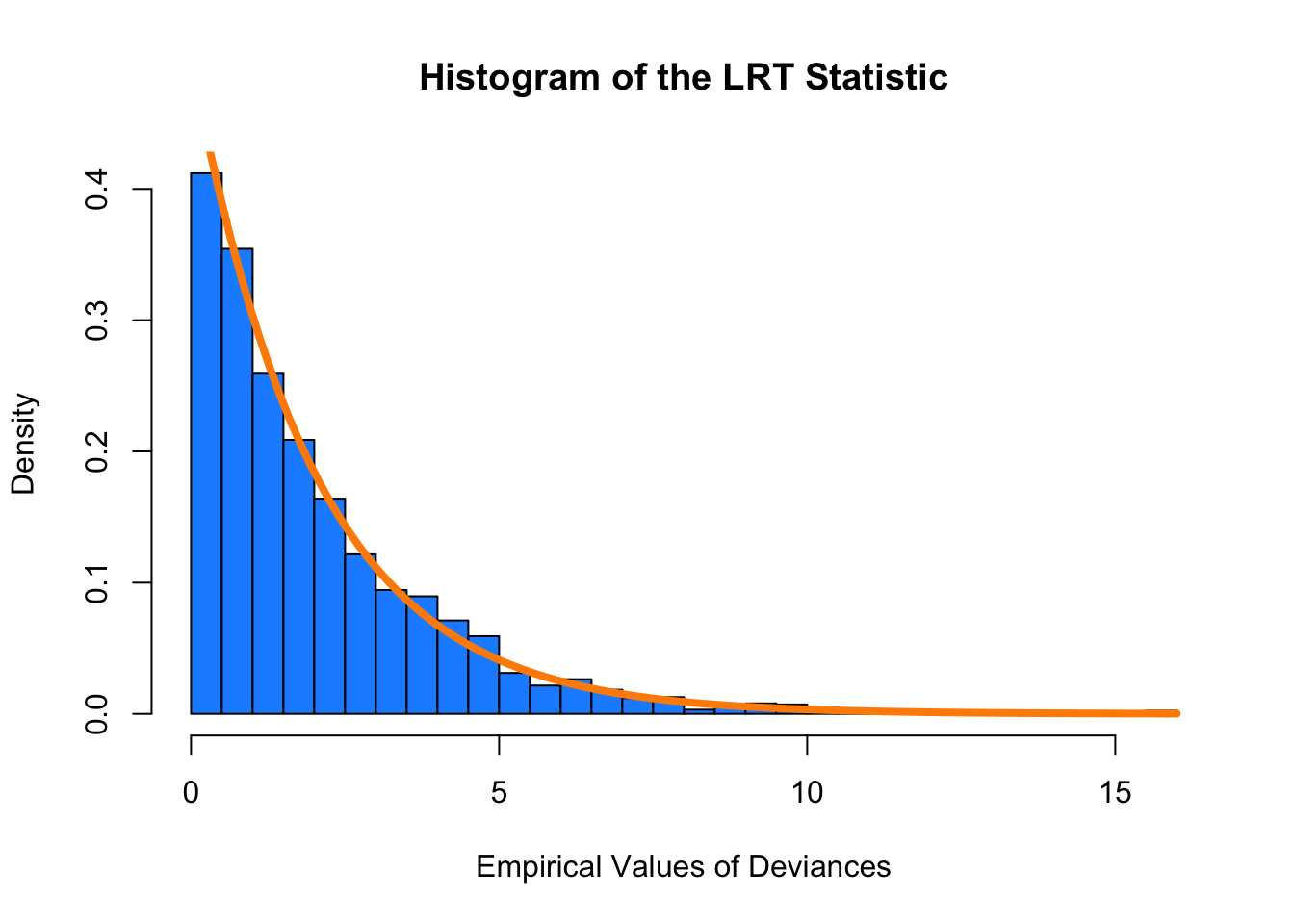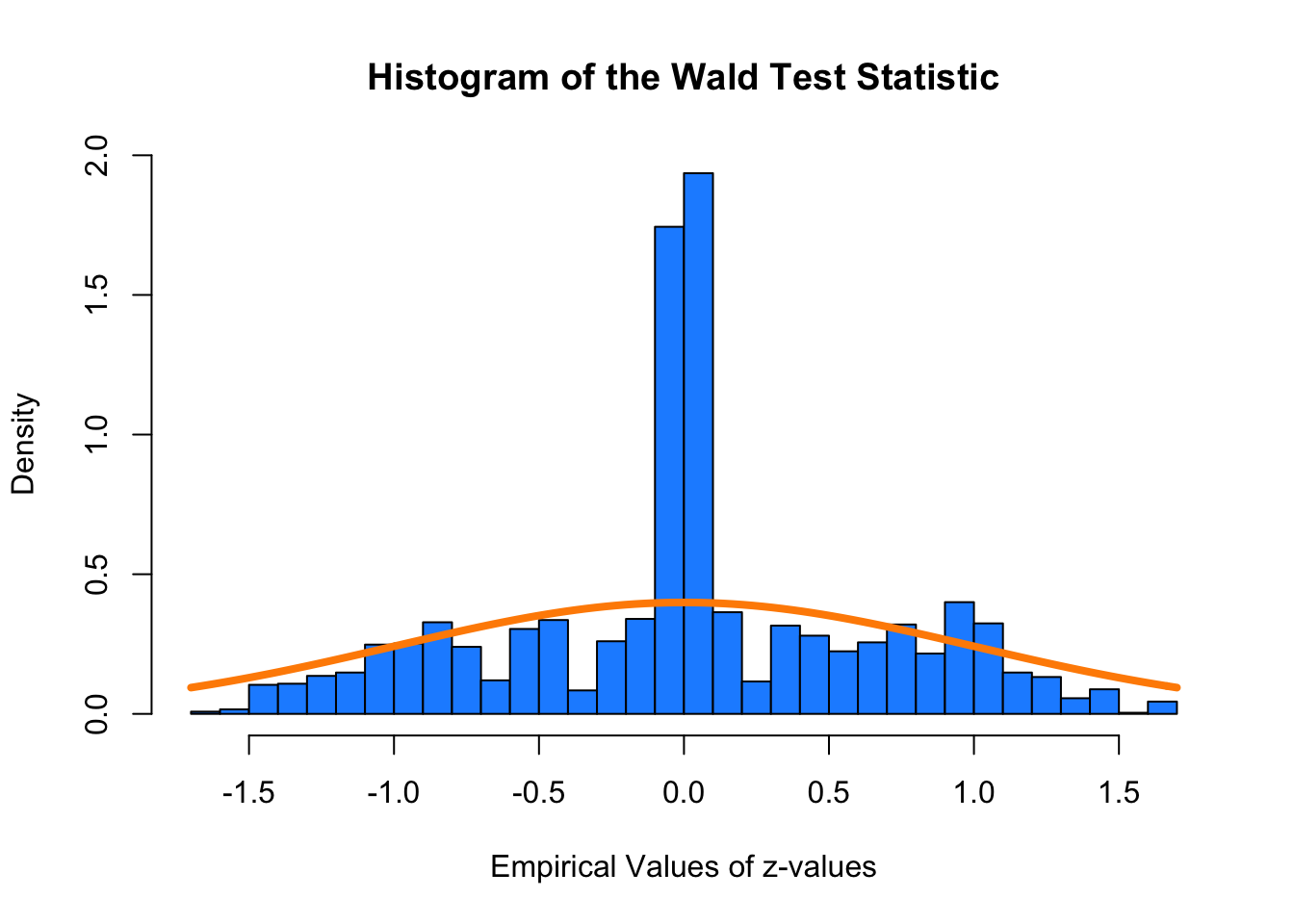## Exercise 1 (Simulating Wald and Likelihood Ratio Tests)

In this exercise we will investigate the distributions of hypothesis tests for logistic regression. For this exercise, we will use the following predictors.

sample_size = 150
set.seed(420)
x1 = rnorm(n = sample_size)
x2 = rnorm(n = sample_size)
x3 = rnorm(n = sample_size)

Recall that

$p({\bf x}) = P[Y = 1 \mid {\bf X} = {\bf x}]$

Consider the true model

$\log\left(\frac{p({\bf x})}{1 - p({\bf x})}\right) = \beta_0 + \beta_1 x_1$

where

• $$\beta_0 = 0.4$$
• $$\beta_1 = -0.35$$

(a) To investigate the distributions, simulate from this model 2500 times. To do so, calculate

$P[Y = 1 \mid {\bf X} = {\bf x}]$

for an observation, and then make a random draw from a Bernoulli distribution with that success probability. (Note that a Bernoulli distribution is a Binomial distribution with parameter $$n = 1$$. There is no direction function in R for a Bernoulli distribution.)

Each time, fit the model:

$\log\left(\frac{p({\bf x})}{1 - p({\bf x})}\right) = \beta_0 + \beta_1 x_1 + \beta_2 x_2 + \beta_3 x_3$

Store the test statistics for two tests:

• The Wald test for $$H_0: \beta_2 = 0$$, which we say follows a standard normal distribution for “large” samples
• The likelihood ratio test for $$H_0: \beta_2 = \beta_3 = 0$$, which we say follows a $$\chi^2$$ distribution (with some degrees of freedom) for “large” samples
beta_0 = 0.4
beta_1 = -0.35
num_sims = 2500
eta = beta_0 + beta_1 * x1
p = 1 / (1 + exp(-eta))

y = rep(0, sample_size)
sim_data = data.frame(y, x1, x2, x3)

wald_test = rep(0, num_sims)
lrt_test = rep(0, num_sims)

for (i in 1:num_sims){
sim_data$y = rbinom(n = sample_size, size = 1, prob = p) mod_full = glm(y ~ x1 + x2 + x3, data = sim_data, family = binomial) mod_1 = glm(y ~ x1, data = sim_data, family = binomial) wald_test[i] = summary(mod_full)$coefficients["x2", "z value"]
lrt_test[i] = anova(mod_1, mod_full, test = "LRT")[2, "Deviance"]
}

(b) Plot a histogram of the empirical values for the Wald test statistic. Overlay the density of the true distribution assuming a large sample.

hist(wald_test, freq = FALSE,
xlab = "Empirical Values of z-values",
main = "Histogram of the Wald Test Statistic",
breaks = 30,
col = "dodgerblue")
x = seq(-1, 1, length = 100)
curve(dnorm(x, mean = 0, sd = 1), col = "darkorange", add = TRUE, lwd = 4)(c) Use the empirical results for the Wald test statistic to estimate the probability of observing a test statistic larger than 1. Also report this probability using the true distribution of the test statistic assuming a large sample.

mean(wald_test > 1)
##  0.168
pnorm(1, mean = 0, sd = 1, lower.tail = FALSE)  
##  0.1587

(d) Plot a histogram of the empirical values for the likelihood ratio test statistic. Overlay the density of the true distribution assuming a large sample.

hist(lrt_test, freq = FALSE,
xlab = "Empirical Values of Deviances",
main = "Histogram of the LRT Statistic",
breaks = 30,
col = "dodgerblue")
k = 3 - 1
curve(dchisq(x, df = k), col = "darkorange", add = TRUE, lwd = 4)(e) Use the empirical results for the likelihood ratio test statistic to estimate the probability of observing a test statistic larger than 5. Also report this probability using the true distribution of the test statistic assuming a large sample.

mean(lrt_test > 5)
##  0.0828
pchisq(5, df = k, lower.tail = FALSE)
##  0.08208

(f) Repeat (a)-(e) but with simulation using a smaller sample size of 10. Based on these results, is this sample size large enough to use the standard normal and $$\chi^2$$ distributions in this situation? Explain.

sample_size = 10
set.seed(420)
x1 = rnorm(n = sample_size)
x2 = rnorm(n = sample_size)
x3 = rnorm(n = sample_size)
beta_0 = 0.4
beta_1 = -0.35
num_sims = 2500
eta = beta_0 + beta_1 * x1
p = 1 / (1 + exp(-eta))

y = rep(0, sample_size)
sim_data_2 = data.frame(y, x1, x2, x3)

wald_test_2 = rep(0, num_sims)
lrt_test_2 = rep(0, num_sims)

for (i in 1:num_sims){
sim_data_2$y = rbinom(n = sample_size, size = 1, prob = p) mod_full = glm(y ~ x1 + x2 + x3, data = sim_data_2, family = binomial) mod_1 = glm(y ~ x1, data = sim_data_2, family = binomial) wald_test_2[i] = summary(mod_full)$coefficients["x2", "z value"]
lrt_test_2[i] = anova(mod_1, mod_full, test = "LRT")[2, "Deviance"]
}

hist(wald_test_2, freq = FALSE,
xlab = "Empirical Values of z-values",
main = "Histogram of the Wald Test Statistic",
breaks = 30,
col = "dodgerblue")
x = seq(-1, 1, length = 100)
curve(dnorm(x, mean = 0, sd = 1), col = "darkorange", add = TRUE, lwd = 4)hist(lrt_test_2, freq = FALSE,
xlab = "Empirical Values of Deviances",
main = "Histogram of the LRT Statistic",
breaks = 30,
col = "dodgerblue")
k = 3 - 1
curve(dchisq(x, df = k), col = "darkorange", add = TRUE, lwd = 4)